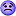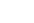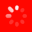The No.1 Website for Pro Audio
All  This Thread  Reviews  Gear Database  Gear for saleLatest  Trending
Here for the gearCan Anyone help with digital EQ algorithms?

Hi all,

I attached a jpg describing a simple cut\boost shelf\band digital equalizer.

I can't really figure out how it is working.

If anyone can share his\hers thoughts i would appreciate the help very much!

Thanks.
Gear Addict

y(n) = alpha*x(n) - alpha*x(n-2) + chi*y(n-1) - beta*y(n-2)

f(x+a) means time shift by a samples, so for implementation you need to save two latest inputs and outputs. The filter is defined by the coefficients alpha, beta and chi. How to obtain them is the hard part.
Here for the geardidn't quite get it

what is the role of alpha and/or beta..?

perhaps you could explain the stages of your equation?
Or relate directly to the graph I posted?
Gear Addict

Quote:
what is the role of alpha and/or beta..?

perhaps you could explain the stages of your equation?
Or relate directly to the graph I posted?
Alpha, beta and such are the filter coefficients. There isn't any intuitive explanation for them. For digital filters they are always real numbers.

I'm not really sure what you want to know, so try to be specific with your questions. For straightforward formulas for implementation there's always http://www.musicdsp.org/files/Audio-EQ-Cookbook.txt
Here for the gear

First of all thank you for your willingness to help.

Basically I'm trying to understand the way a digital EQ is performing its' boosts or cuts.
I thought that generally this is achieved by a designed IR which is convolved with the i/p signal to produce the o/p signal.
I guess in this algorithm this is not the case.
So how does it work then?
What are the stages in this algorithm?
Gear Addict

Quote:
First of all thank you for your willingness to help.

Basically I'm trying to understand the way a digital EQ is performing its' boosts or cuts.
I thought that generally this is achieved by a designed IR which is convolved with the i/p signal to produce the o/p signal.
I guess in this algorithm this is not the case.
So how does it work then?
What are the stages in this algorithm?
With an infinite impulse response you can't use convolution, because it would require an infinite amount of time. With FIR filters you can do that.

Maybe it clarifies to follow what happens to an impulse.
the input is: x(0) is 1 and all other x(n) are zero.
y(n) is the output.
y(0) = alpha*x(0) - alpha*x(-2) + chi*y(-1) - beta*y(-2) = alpha*1 because other parts of the sum are zero
y(1) = alpha*x(1) - alpha*x(-1) + chi*y(0) - beta*y(-1) = chi*alpha*1
y(2)= alpha*x(2) - alpha*x(0) + chi*y(1) - beta*y(0) = -alpha*1 + chi*chi*alpha*1 - beta*alpha*1

From this on it gets only more messy but the principle is the same. If you want to know why it works, you may be out of luck, that requires knowledge on complex analysis and z-transform. But you may think of it as an algorithmical way to replace convolution and a stored impulse response.
Gear Maniac

Sorry to interrupt, but what level of math is required to understand what you guys are talking about?
Here for the gear

obviously you need a decent level of math to approach this stuff.

jdtrbn i still didn't understand your expanation.
perhaps you can guide me through the equation in words..? i don't really understand what is "chi", or the role of alpha and beta.

will appreciate it a lot.

thanks.
Gear Addict

Quote:
obviously you need a decent level of math to approach this stuff.
For physics and math students complex analysis and fourier transforms were second year maths in our university.
Quote:
jdtrbn i still didn't understand your expanation.
perhaps you can guide me through the equation in words..? i don't really understand what is "chi", or the role of alpha and beta.
Chi is the name of the greek letter on the right. The equation is simply the sum (letter sigma) shown in the graph written down. An empty triangle means multiplying by the coefficient that is shown, so I've just written these products and added them, as after multiplying there are arrows pointing at sigma. From sigma there's an arrow to y(n) so this is the output.

I don't know what you mean by a "role", they are constants that are used for multiplying stuff and there isn't any intuitive explanation for them.
Gear Addict

if you want to get a full understanding of how filters work, how to construct or design them, I can highly recommend the book 'The Scientist and Engineer's Guide to Digital Signal Processing'.
You can look at the chapters online, for free at The Scientist and Engineer's Guide to Digital Signal Processing

Peter

## Welcome to the Gearslutz Pro Audio Community!

###### Registration benefits include:
• The ability to reply to and create new discussions
• Access to members-only giveaways & competitions
• Interact with VIP industry experts in our guest Q&As
• Access to members-only sub forum discussions
• Access to members-only Chat Room
• Get INSTANT ACCESS to the world's best private pro audio Classifieds for only USD \$20/year
• Promote your eBay auctions and Reverb.com listings for free
• Remove this message!
You need an account to post a reply. Create a username and password below and an account will be created and your post entered.Slide to join now Processing…
Thread Tools
Search this Thread
Search this Thread:

Advanced Search
Forum Jump
Forum Jump